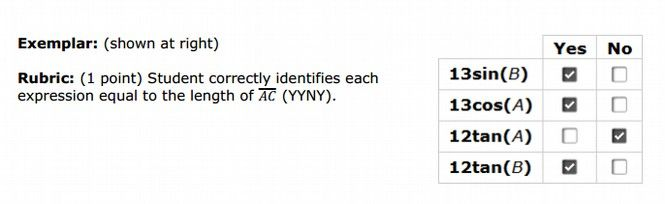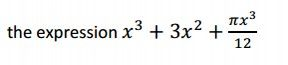You are here:   Home > End the Unfair SBAC Test > SBAC 11th Grade Practice Math Test ANSWERS

Here are the answers to our own SBAC Practice Test:

#6
ANSWER: 55, 65, 75 and 85.

#7 ANSWER: Seven is a tricky question. It says to add “a” point. In plain English this would mean a single point. It fact, you must click on THREE points (-2,3), (0,2) and (2.5, 0)

#8 ANSWER: The key claims you should only check the second and fourth boxes. However, it seems to me that there are values of X that result in an integer solution for all 5 boxes!

#9 ANSWER: The answer is 5. I think this was a very difficult problem.

#13 ANSWER: First box: (17 + 2w)(13 + 2w) = 396
Second box: w = 5/2

#15 ANSWER: The key says the answer is 1 to 1.4. In fact, it is 1 centimeter per day.

#20 ANSWER: 2t + 3t = 24

#21 ANSWER: Tina is incorrect because some orders of basic transformations do not produce the same results. Suppose we move triangle A 2 units to the right first. The point (4, 3) is then (6, 3). Then, we take the reflection across the x-axis, which makes that point (6, -3). A reflection of (6, -3) across the y-axis gives us (-6, -3), which is not one of the vertices of triangle A’. Therefore, the basic transformations done in any order do not produce the same result.

#26 ANSWER: Key says 3 in first box and -2 in second box. But there are many possible right answers and many possible wrong answers so how can this question even be graded?#29 ANSWER: B, C, D and F.